# Graph imbedding

(diff) ← Older revision | Latest revision (diff) | Newer revision → (diff)

A mapping of the vertices and edges of a graph into points and continuous curves of a given space, respectively, such that the vertices incident to an edge are mapped to the end points of the curve corresponding to this edge. A regular imbedding is an imbedding in which different points correspond to different vertices while curves corresponding to edges do not pass (except for their terminal points) through points corresponding to the vertices and do not intersect. Any graph can be regularly imbedded in three-dimensional space. A graph that can be regularly imbedded in a plane is said to be planar. There exist non-planar graphs, such as the graphs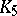and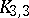(cf. Graph, planar, Figure 1). The smallest genus of the two-dimensional orientable surface in which a graphcan be regularly imbedded is said to be the genus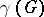of. It has been proved, in particular, that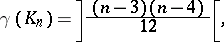where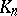is the complete graph withvertices and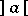is the least integer not smaller than;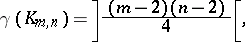where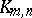is the complete bipartite graph (cf. Graph, bipartite);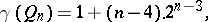where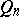is the-dimensional cube. The thickness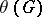of a graphis the smallest number of its planar subgraphs whose union yields. It has been proved, in particular, that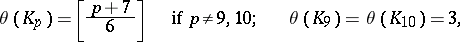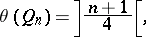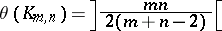(possibly with some exceptions).

Other numerical characteristics connected with graph imbeddings have also been studied. These include, for example, the number of crossings — the smallest number of intersections of edges sufficient to imbed a given graph in a given surface; the coarseness — the largest number of non-planar subgraphs in a given graph which do not have common edges, etc. Imbeddings in non-orientable surfaces have also been considered. An imbedding of a graph in an-dimensional integral lattice is a mapping into the lattice under which the vertices are mapped to different nodes of the lattice, while the edges follow the lattice lines.

Problems involving graph imbedding in surfaces and in lattices occur in automatic computer design, communication design, etc.

How to Cite This Entry:
Graph imbedding. Encyclopedia of Mathematics. URL: http://encyclopediaofmath.org/index.php?title=Graph_imbedding&oldid=18375
This article was adapted from an original article by V.B. Alekseev (originator), which appeared in Encyclopedia of Mathematics - ISBN 1402006098. See original article